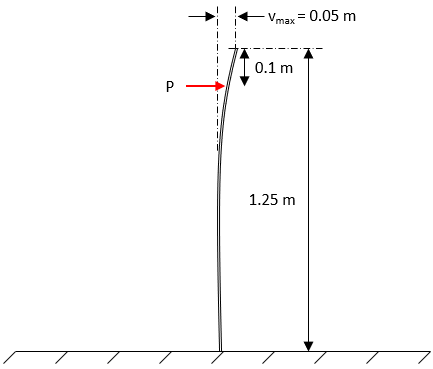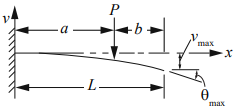## Vertically Cantilevered

Consider a 1.25 m long solid aluminum rod with a round cross section is vertically cantilevered in the ground with a force P applied horizontally 0.1 m below the free end as shown. If there is a max deflection of 0.05 m at the rod’s free end, calculate the required force. Note the Modulus of Elasticity is 70 GPa, and the cross-sectional moment of inertia is 2.00∙10^(-8)m^4.Hint
The deflection formula for this type of cantilevered beam problem is:
$$v_{max}=\frac{Pa^2}{6EI}(3L-a)$$$where $$E$$ is the Modulus of Elasticity $$L$$ is the beam’s length, $$P$$ is the force, and $$I$$ is the moment of inertia. Hint 2The deflection formula for this type of cantilevered beam problem is: $$v_{max}=\frac{Pa^2}{6EI}(3L-a)$$$
where $$E$$ is the Modulus of Elasticity $$L$$ is the beam’s length, $$P$$ is the force, $$I$$ is the moment of inertia, and $$a$$ is:Solving for the force, $$P$$ :
$$P=\frac{v_{max}(6EI)}{(3L-a)(a^2)}$$$$$P=\frac{(0.05m)(6)(70\cdot 10^9\frac{N}{m^2})(2\cdot 10^{-8}m^4)}{[(3)(1.25m)-(1.25m-0.1m)](1.25m-0.1m)^2}$$$
$$=\frac{420N\cdot m^3}{(3.75m-1.15m)(1.15m)^2}=\frac{420N\cdot m^3}{(3.75m-1.15m)(1.15m)^2}$$$$$=\frac{420N\cdot m^3}{(2.6m)(1.323m^2)}=122.15\:N$$$
122.15 N